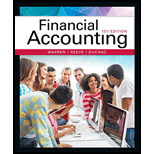# Equipment with a cost of $180,000 has an estimated residual value of$14,400, has an estimated useful life of 16 years, and is depreciated by the straight-line method. (a) Determine the amount of the annual depreciation. (b) Determine the book value at the end of the tenth year of use. (c) Assuming that at the start of the eleventh year the remaining life is estimated to be eight years and the residual value is estimated to be $10,500, determine the depreciation expense for each of the remaining eight years.BuyFindarrow_forward ### Financial Accounting 15th Edition Carl Warren + 2 others Publisher: Cengage Learning ISBN: 9781337272124 #### Solutions Chapter SectionBuyFindarrow_forward ### Financial Accounting 15th Edition Carl Warren + 2 others Publisher: Cengage Learning ISBN: 9781337272124 Chapter 10, Problem 4PEA Textbook Problem 191 views ## Equipment with a cost of$180,000 has an estimated residual value of $14,400, has an estimated useful life of 16 years, and is depreciated by the straight-line method. (a) Determine the amount of the annual depreciation. (b) Determine the book value at the end of the tenth year of use. (c) Assuming that at the start of the eleventh year the remaining life is estimated to be eight years and the residual value is estimated to be$10,500, determine the depreciation expense for each of the remaining eight years.

(a)

To determine

Determine the amount of annual depreciation

### Explanation of Solution

Straight-line Depreciation: Under the straight-line method of depreciation, the same amount of depreciation is allocated every year over the estimated useful life of an asset. The formula to calculate the depreciation cost of the asset using the salvage value is shown as below:

Depreciation cost = (Cost of the asset-residual value)Estimated useful life of the asset

Determine the amount of annual depreciation.

Cost of the equipment =$180,000 Residual Value =$14,400

Estimated Useful life =16 years

AnnualD

(b)

To determine

Determine the book value at the end of the tenth year of use.

(c)

To determine

Determine the revised annual depreciation.

### Still sussing out bartleby?

Check out a sample textbook solution.

See a sample solution

#### The Solution to Your Study Problems

Bartleby provides explanations to thousands of textbook problems written by our experts, many with advanced degrees!

Get Started

Find more solutions based on key concepts
What are the differences between Internal and external recruiting?

Foundations of Business (MindTap Course List)

(Appendix 4B) Describe the difference between producing and support departments.

Managerial Accounting: The Cornerstone of Business Decision-Making

INFLATION CROSS-PRODUCT An analyst is evaluating securities in a developing nation where the inflation rate is ...

Fundamentals of Financial Management, Concise Edition (with Thomson ONE - Business School Edition, 1 term (6 months) Printed Access Card) (MindTap Course List)